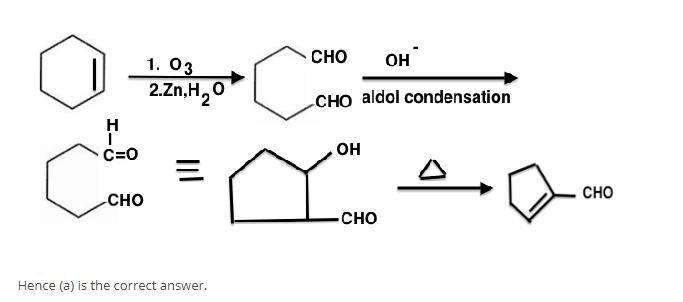# JEE(MAIN) Chemistry Mock Test - 2

## 30 Questions MCQ Test JEE Main Mock Test Series 2021 & Previous Year Papers | JEE(MAIN) Chemistry Mock Test - 2

Description
This mock test of JEE(MAIN) Chemistry Mock Test - 2 for JEE helps you for every JEE entrance exam. This contains 30 Multiple Choice Questions for JEE JEE(MAIN) Chemistry Mock Test - 2 (mcq) to study with solutions a complete question bank. The solved questions answers in this JEE(MAIN) Chemistry Mock Test - 2 quiz give you a good mix of easy questions and tough questions. JEE students definitely take this JEE(MAIN) Chemistry Mock Test - 2 exercise for a better result in the exam. You can find other JEE(MAIN) Chemistry Mock Test - 2 extra questions, long questions & short questions for JEE on EduRev as well by searching above.
QUESTION: 1

### The compound 'A' when treated with methyl alcohol and few drops of H₂SO₄ give wintergreen smell. The compound 'A' is

Solution: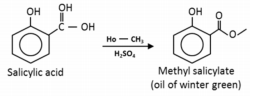QUESTION: 2

### Which of the following will not give iodoform test?

Solution: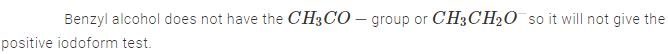QUESTION: 3

### phospholipids are esters of glycerol with

Solution: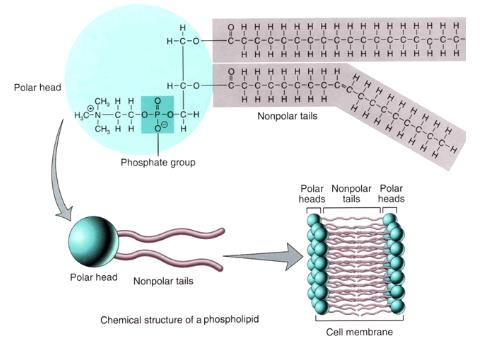QUESTION: 4

In graphite, the electrons are

Solution: Carbon has four spare electrons.Electron that forms pi bond is freer to travel between atoms compared to the others and is theoretically associated with all adjacent atoms (imagine the 3d structure of graphite) rather than a single atom. Because of that, it is called delocalized electron.
QUESTION: 5

The maximum number of unpaired d-electrons are in

Solution:

Fe+3 has 5 unpaired electrons, more than other ones.

QUESTION: 6

Splitting of spectral lines under the influence of magnetic field is called

Solution:

In stark effect spectral lines split due to electricity.
In photoelectric effect the splitting of spectral line takes place as the light falls on the metal.

QUESTION: 7

Alkyl groups are o- and p-directing because of

Solution:
QUESTION: 8

In which of the following molecule, all the atoms lie in one plane?

Solution:

It has got sp2 hybridization, giving it a planar structure.Further backbonding from flouine makes the B-F bonds stronger than normal single bonds

QUESTION: 9

Pairs of species having identical shapes for molecules is

Solution:
Explanation:
XeF2 contains sp3d hybridised Xe atom with three lone pairs of electrons. Fluorine atoms occupy axial positions while three lone pairs of electrons occupy equatorial positions in trigonal bipyramid. Since the lone pair of electrons does not consider for the shape of the molecule, thus, the shape of XeF2 comes out to be linear. While C -atom of CO2 is sp hybridised and thus, possesses a linear shape.QUESTION: 10

Which one of the following is expected to exhibit optical isomerism

Solution:
QUESTION: 11

Nickel is purified by

Solution: The Mond process, sometimes known as the carbonyl process, is a technique created by Ludwig Mond in 1890, to extract and purify nickel. The process was used commercially before the end of the 19th century. This process converts nickel oxides into pure nickel.
QUESTION: 12

The oxidation state of Cr in [Cr(NH₃)₄Cl₂]⁺ is

Solution:

Applying sum of individual charge = charge on molecule
x+(0)×4 + (-1)×2 = +1
x=3

QUESTION: 13

Which of the following noble gas is least polarisable?

Solution: Although this applies to all noble gases, except helium. There are two electrons moving around the nucleus. The movement of those electrons is symmetrical and during a defined amount of time, the atom is not polarised. However, the momentary positions of those electrons can polarise the atom. This can happen when both electrons are relatively close, on the same one side of the atom. In this case a momentary dipole is formed, since there is separation of two charges, nucleus being positive and the pair of electrons being the negative pole.Hence, He has least polarized.
QUESTION: 14

The IUPAC name of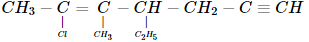Solution:

Correct name is 2-Chloro-4-ethyl-3-methyl hept-2-ene-6-yne

QUESTION: 15

When HCl gas is passed through a saturated solution of common salt, pure NaCl is precipitated because

Solution:
QUESTION: 16

Which of the following pair represents stereoisomerism?

Solution:
QUESTION: 17

Which of the following pair of elements belongs to same period of the periodic table?

Solution:
QUESTION: 18

Natural rubber is a polymer of

Solution:
QUESTION: 19

In view of their low ionization energies, the alkali metals are

Solution: Strong reducing agents as they can loose the electrons very easily.
QUESTION: 20

100 ml of a liquid A was mixed with 25 ml of a liquid B to give B to give a non-ideal solution of A-B mixture. The volume of this mixture would be

Solution:

Concept: Negative deviation. Final volume decreases due to negative deviation  from original volume 125 ml. So, the volume of the mixture would be close to 125 but not exact 125. Hence, (c) is the correct answer.

QUESTION: 21

Ethylbenzene with Br2 in the presence of FeBr3 gives

Solution:

C2H5 ethyl group is electron donating group , and when an electron donating group is attached to the benzene ring it increases affinity of other molecules to get attached at the ortho and para positions .

QUESTION: 22

Geometric isomerism will be exhibited by

Solution:

In this compound both the carbon atoms bonded by the double bond have two different group attached to them

QUESTION: 23

Formation of ammonia from H₂ and N₂ by Haber's process using Fe is an example of

Solution: If reactants and catalyst are not in the same phase then it is called heterogeneous catalysis here Fe is solid, N2 is gas.
QUESTION: 24

Which of the following transition metal cation has maximum unpaired electrons ?

Solution:
QUESTION: 25

Among the following, the surfactant that will form micelles in aqueous solution at the lowest molar concentration at ambient conditions is

Solution:

The CMC (critical micelle concentration) value of the surfactants depends both on the size of the hydrophobic chain (tail) and size of the hydrophilic domain (head). The two contributions are counteracting with a lower CMC for a larger hydrophobic chain and a higher hydrophilic domain.

QUESTION: 26

The percentage of p-character in the orbitals forming P ― P bonds in P4 is

Solution: The percentage of p-character in forming P-P bonds in P4 molecule is: 0. In forming P4molecule, P atom uses sp3 hybrid orbitals. Thus, p-character in hybrid orbitals is 75%.
QUESTION: 27

How many bonds are there in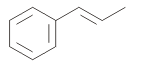Solution:

It's C(i.e.19sigma and 4π bond.)

QUESTION: 28

The number of structural isomers for C6H14 is

Solution:
QUESTION: 29

Among the following, the coloured compound is

Solution:

In CuF2, Cu2+ ions exist, having de configuration.

Unpaired electron causes colour (d-d transition).

In the crystalline form, CuF2 is blue coloured.

QUESTION: 30

Cyclohexene on ozonolysis followed by reaction with zinc dust and water gives compound E. Compound E on further treatment with aqueous KOH yields compound F. Compound F is

Solution: